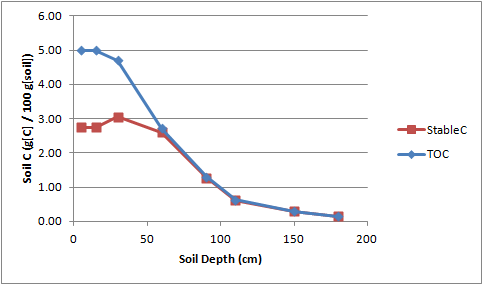# agmip.github.io

AgMIP open source software development and use.

View the Project on GitHub

# STABLEC - Stable C fraction distribution in soil layers

### Inputs:

• SOM3_0 = fraction of total soil organic C which is stable, at surface (fraction)
• PP = depth of topsoil where maximum SOM3 fraction is relatively constant (cm)
• RD = depth at which soil C is relatively stable (~98% stable C) (cm)

### Implicit Inputs (i.e., available in the data):

• SLLB = array of depths to bottom of soil layer (cm)
• SLOC = array of total organic C (g[C]/100 g[soil])

### Outputs:

• SLSC = array of Stable C (g[C]/100g[soil])

### Procedure:

1. Calculate SOM2 fraction at surface
``````SOM2_0 = 0.95 * (1 - SOM3_0)
``````
2. Call Root_dist function with PP, M=SOM2_0, RD, returns F
3. Calculate array SOM2_frac(j) = MAX (0.02, F(j))
4. Calculate array SOM3_frac(j) = 1 - SOM2_frac(j) / 0.95
5. Calculate array StableC(j) = TOC(j) * SOM3_frac(j)

### Example:

``````FILL, SLSC, STABLEC(), 0.55, 20, 60
``````

#### Input:

``````TOC_0 = 5 (g[C]/100 g[soil])
SOM3_0 = 0.55 (fraction of TOC)
PP = 20 (cm)
RD = 60 (cm)
``````

#### Root distribution function parameters:

``````M = 0.4275
PP = 20
RD = 60
k = -0.0978
``````

#### Calculation of stable C:

sllb TOC mid F SOM2_frac SOM3_frac StableC
5 5.00 2.5 0.428 0.428 0.550 2.75
15 5.00 10 0.428 0.428 0.550 2.75
30 4.70 22.5 0.335 0.335 0.648 3.05
60 2.71 45 0.037 0.037 0.961 2.61
90 1.30 75 0.002 0.020 0.979 1.28
110 0.63 100 0.000 0.020 0.979 0.61
150 0.30 130 0.000 0.020 0.979 0.29
180 0.14 165 0.000 0.020 0.979 0.14### More Samples:

The following DOME spreadsheet is using this function. Machakos Overlay DOME

Dome functions page

Home